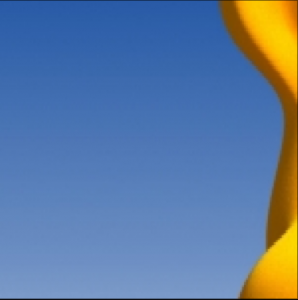Related Articles
Python PIL | Image.resize() method
• Last Updated : 17 Jul, 2019

PIL is the Python Imaging Library which provides the python interpreter with image editing capabilities. The `Image` module provides a class with the same name which is used to represent a PIL image. The module also provides a number of factory functions, including functions to load images from files, and to create new images.

`Image.resize()` Returns a resized copy of this image.

Syntax: Image.resize(size, resample=0)
Parameters:

size – The requested size in pixels, as a 2-tuple: (width, height).
resample – An optional resampling filter. This can be one of PIL.Image.NEAREST (use nearest neighbour), PIL.Image.BILINEAR (linear interpolation), PIL.Image.BICUBIC (cubic spline interpolation), or PIL.Image.LANCZOS (a high-quality downsampling filter). If omitted, or if the image has mode “1” or “P”, it is set PIL.Image.NEAREST.

Returns type: An Image object.

Image Used:`  ` `# Improting Image class from PIL module ``from` `PIL ``import` `Image `` ` `# Opens a image in RGB mode ``im ``=` `Image.``open``(r``"C:\Users\System-Pc\Desktop\ybear.jpg"``) `` ` `# Size of the image in pixels (size of orginal image) ``# (This is not mandatory) ``width, height ``=` `im.size `` ` `# Setting the points for cropped image ``left ``=` `4``top ``=` `height ``/` `5``right ``=` `154``bottom ``=` `3` `*` `height ``/` `5`` ` `# Cropped image of above dimension ``# (It will not change orginal image) ``im1 ``=` `im.crop((left, top, right, bottom))``newsize ``=` `(``300``, ``300``)``im1 ``=` `im1.resize(newsize)``# Shows the image in image viewer ``im1.show() `

Output:Another example:Here we use the different newsize value.

 `# Improting Image class from PIL module ``from` `PIL ``import` `Image `` ` `# Opens a image in RGB mode ``im ``=` `Image.``open``(r``"C:\Users\System-Pc\Desktop\ybear.jpg"``) `` ` `# Size of the image in pixels (size of orginal image) ``# (This is not mandatory) ``width, height ``=` `im.size `` ` `# Setting the points for cropped image ``left ``=` `6``top ``=` `height ``/` `4``right ``=` `174``bottom ``=` `3` `*` `height ``/` `4`` ` `# Cropped image of above dimension ``# (It will not change orginal image) ``im1 ``=` `im.crop((left, top, right, bottom))``newsize ``=` `(``200``, ``200``)``im1 ``=` `im1.resize(newsize)``# Shows the image in image viewer ``im1.show() `

Output:Attention geek! Strengthen your foundations with the Python Programming Foundation Course and learn the basics.

To begin with, your interview preparations Enhance your Data Structures concepts with the Python DS Course.

My Personal Notes arrow_drop_up